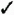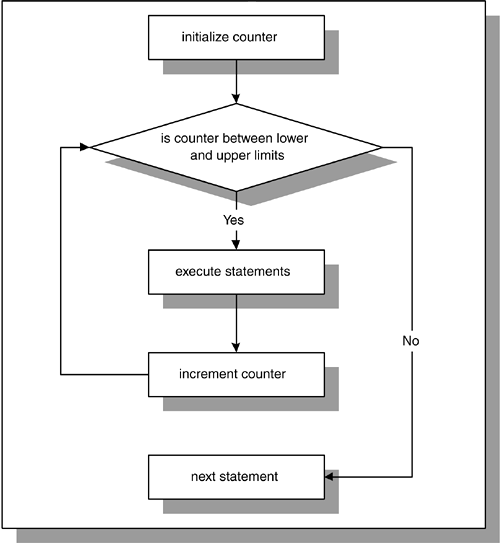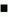# Lab 8.3 Numeric FOR Loops

## Lab Objectives

After this Lab, you will be able to:Use Numeric FOR Loops with the IN OptionUse Numeric FOR Loops with the REVERSE Option

A numeric FOR loop is called numeric because it requires an integer as its terminating value. Its structure is as follows :

` `
` FOR loop_counter IN[REVERSE] lower_limit..upper_limit LOOP    STATEMENT 1;    STATEMENT 2;    ...    STATEMENT N; END LOOP; `

The reserved word FOR marks the beginning of a FOR loop construct. The variable, loop_counter , is an implicitly defined index variable. There is no need to define the loop counter in the declaration section of the PL/SQL block. This variable is defined by the loop construct. Lower_limit and upper_limit are two integer numbers that define the number of iterations for the loop. The values of the lower_limit and upper_limit are evaluated once, for the first iteration of the loop. At this point, it is determined how many times the loop will iterate. Statements 1 through N are a sequence of statements that is executed repeatedly. END LOOP is a reserved phrase that marks the end of the loop construct.

The reserved word IN or IN REVERSE must be present when defining the loop. If the REVERSE keyword is used, the loop counter will iterate from the upper limit to the lower limit. However, the syntax for the limit specification does not change. The lower limit is always referenced first. The flow of this logic is illustrated in Figure 8.4.

##### Figure 8.4. Numeric FOR LoopFigure 8.4 shows that the loop counter is initialized to the lower limit for the first iteration of the loop only. However, the value of the loop counter is tested for each iteration of the loop. As long as the value of v_counter ranges from the lower limit to the upper limit, the statements inside the body of the loop are executed. When the value of the loop counter does not satisfy the range specified by the lower limit and the upper limit, control is passed to the first executable statement outside the loop.FOR EXAMPLE

` `
` BEGIN    FOR v_counter IN 1..5 LOOP       DBMS_OUTPUT.PUT_LINE ('v_counter = 'v_counter);    END LOOP; END; `

In this example, there is no declaration section for the PL/SQL block because the only variable used, v_counter , is the loop counter. Numbers 1..5 specify the range of the integer numbers for which this loop is executed.

Notice that there is no statement

` `
`  v_counter := v_counter + 1  `

anywhere , inside or outside the body of the loop. The value of v_counter is incremented implicitly by the FOR loop itself.

This example produces the following output when run:

` `
`  v_counter = 1   v_counter = 2   v_counter = 3   v_counter = 4   v_counter = 5   PL/SQL procedure successfully completed.  `

As a matter of fact, if you include the statement

` `
`  v_counter := v_counter + 1  `

in the body of the loop, the PL/SQL script will compile with errors. Consider the following example.FOR EXAMPLE

` `
` BEGIN    FOR v_counter IN 1..5 LOOP       v_counter := v_counter + 1;       DBMS_OUTPUT.PUT_LINE ('v_counter = ' v_counter);    END LOOP; END; `

When this example is run, the following error message is produced:

` `
`  BEGIN   *   ERROR at line 1:   ORA-06550: line 3, column 7:   PLS-00363: expression 'V_COUNTER' cannot be used as an   assignment target   ORA-06550: line 3, column 7:   PL/SQL: Statement ignored  `It is important to remember that the loop counter is implicitly defined and incremented when a numeric FOR loop is used. As a result, it cannot be referenced outside the body of the FOR loop. Consider the following example: ` ` ` BEGIN FOR v_counter IN 1..5 LOOP DBMS_OUTPUT.PUT_LINE ('v_counter = 'v_counter); END LOOP; DBMS_OUTPUT.PUT_LINE ('Counter outside the loop is ' v_counter); END; ` When this example is run, the following error message is produced: ` ` ` DBMS_OUTPUT.PUT_LINE ('Counter outside the loop is ' v_counter); * ERROR at line 5: ORA-06550: line 5, column 53: PLS-00201: identifier 'V_COUNTER' must be declared ORA-06550: line 5, column 4: PL/SQL: Statement ignored ` Because the loop counter is declared implicitly by the loop, the variable v_counter cannot be referenced outside the loop. As soon as the loop completes, the loop counter ceases to exist.

#### Using the REVERSE Option in the Loop

Earlier in this section, you encountered two options that are available when the value of the loop counter is evaluated, IN and IN REVERSE. You have seen examples already that demonstrate the usage of the IN option for the loop. The next example demonstrates the usage of the IN REVERSE option for the loop.FOR EXAMPLE

` `
` BEGIN    FOR v_counter IN REVERSE 1..5 LOOP       DBMS_OUTPUT.PUT_LINE ('v_counter = 'v_counter);    END LOOP; END; `

When this example is run, the following output is produced:

` `
`  v_counter = 5   v_counter = 4   v_counter = 3   v_counter = 2   v_counter = 1   PL/SQL procedure successfully completed.  `

As mentioned before, even though the REVERSE keyword is present, the lower limit of the loop is referenced first. However, it is important to note that the loop counter is evaluated from the upper limit to the lower limit. For the first iteration of the loop, v_counter (in our case it is a loop counter) is initialized to 5 (upper limit). Then its value is displayed on the screen. For the second iteration of the loop, the value of v_counter is decreased by 1, and displayed on the screen.

Notice that the number of times the body of the loop is executed is not affected by the option used, IN or IN REVERSE. Only the values assigned to the lower limit and the upper limit determine how many times the body of the loop is executed.

#### Premature Termination of the Loop

The EXIT and EXIT WHEN statements can be used inside the body of a numeric FOR loop. If the EXIT condition evaluates to TRUE before the loop counter reaches its terminal value, the FOR loop is terminated prematurely. If the loop counter reaches its terminal value before the EXIT condition yields TRUE, there is no premature termination of the FOR loop. Consider the following:

` `
` FOR LOOP_COUNTER IN LOWER_LIMIT..UPPER_LIMIT LOOP    STATEMENT 1;    STATEMENT 2;    IF  EXIT_CONDITION  THEN       EXIT;    END IF; END LOOP; STATEMENT 3; `

or

` `
` FOR LOOP_COUNTER IN LOWER_LIMIT..UPPER_LIMIT LOOP    STATEMENT 1;    STATEMENT 2;    EXIT WHEN  EXIT_CONDITION  ; END LOOP; STATEMENT 3; `

Consider the following example of a FOR loop that uses the EXIT WHEN condition. This condition is causing the loop to terminate prematurely.FOR EXAMPLE

` `
` BEGIN    FOR v_counter IN 1..5 LOOP       DBMS_OUTPUT.PUT_LINE ('v_counter = 'v_counter);       EXIT WHEN v_counter = 3;    END LOOP; END; `

Notice that according to the range specified, the loop should execute five times. However, the loop is executed only three times because the EXIT condition is present inside the body of the loop. Thus, the loop terminates prematurely.Oracle PL[s]SQL by Example
ISBN: 3642256902
EAN: N/A
Year: 2003
Pages: 289